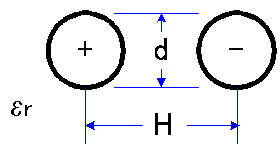## Open Wire Line Impedance Calculator

1. Choose the line structure to be calculated, and then fill in the relative permittivity of the dielectric and the dimension parameters.
2. Select the Z0 button to calculate the characteristic impedance of the corresponding line.

If you leave the relative permittivity Er blank, a default line dimension will be used, and the characteristic impedance is calculated after selecting the Z0 button. Equations used to make these calculations can be found in the book , and are also provided below the corresponding structure.

1. Single wire above ground

 Parameters: erH mm d mmCalculation Results: ohms2. Balanced two-wire line

 Parameters: erH mm d mmCalculation Results: ohmsK. Chang, "Handbook of Microwave and Optical Components", Vol 1,John Wiley & Sons, New York, p. 8, 1989.

THE FORMULAS ARE APPROXIMATIONS!
They should not be used when a high degree of accuracy is required.
This calculator is developed by Kami Electromagnetic Compatibility Laboratory, UEC. Questions concerning this calculator or update information for this web site should be sent to